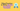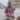# 5 Best Practices to Write Quality JavaScript Variables

Posted December 1, 2020Start discussion

Variables are everywhere. Even if you’re writing a small function, or writing an application: you always declare, assign, and read variables.

Writing quality variables brings increased readability and easier maintainability of your code.

In this post, you’ll read 5 best practices of how to declare and use variables in JavaScript.

## 1. Prefer const, otherwise use let

I declare my JavaScript variables using `const` or `let`.

The main difference between the two is that `const` variable requires an initial value, and its value can not be reassigned once initialized.

`javascript`// const requires initializationconst pi = 3.14;// const cannot be reassignedpi = 4.89; // throws "TypeError: Assignment to constant variable"``

`let` declaration, on the other side, doesn’t require an initial value and you can reassign its value multiple times.

`javascript`// let initialization is optionallet result;// let can be reassignedresult = 14;result = result * 2;``

`const` is a one-off assignment variable. Reasoning about a `const` variable is easier (compared to `let`) because you know that a `const` variable isn’t going to be changed.

A good practice when choosing the declaration type of variables is to prefer `const`, otherwise use `let`.

For example, if you’re looking at a function body and see a `const result = ...` declaration:

`javascript`function myBigFunction(param1, param2) {  /* lots of stuff... */  const result = otherFunction(param1);  /* lots of stuff... */  return something;}``

Without knowing what happens inside `myBigFunction()`, you can conclude that `result` variable is assigned once and after the declaration is read-only.

In other cases, if your variable has to be reassigned multiple times during execution, then `let` declaration is the way to go.

## 2. Minimize the variable’s scope

The variables live within the scope they’ve been created. A code block and a function body create a scope for `const` and `let` variables.

A good practice to increase the readability of variables is to keep them in the smallest scope.

For example, the following function is an implementation of binary search algorithm:

`javascript`function binarySearch(array, search) {  let middle;  let middleItem;  let left = 0;  let right = array.length - 1;  while(left <= right) {    middle = Math.floor((left + right) / 2);    middleItem = array[middle];    if (middleItem === search) {       return true;     }    if (middleItem < search) {       left = middle + 1;     } else {      right = middle - 1;     }  }  return false;}binarySearch([2, 5, 7, 9], 7); // => truebinarySearch([2, 5, 7, 9], 1); // => false``

The `middle` and `middleItem` variables are declared at the beginning of the function body. Thus, these variables are available within the entire scope created by `binarySearch()` function body.

`middle` variable keeps the middle index of binary search, while `middleItem` variable keeps the middle item.

However, `middle` and `middleItem` variables are used only within the `while` cycle code block. So… why not declaring these variables directly within `while` code block?

`javascript`function binarySearch(array, search) {  let left = 0;  let right = array.length - 1;  while(left <= right) {    const middle = Math.floor((left + right) / 2);    const middleItem = array[middle];    if (middleItem === search) {      return true;     }    if (middleItem < search) {      left = middle + 1;     } else {      right = middle - 1;     }  }  return false;}``

Now, `middle` and `middleItem` variables exist solely in the scope that uses the variables. They have a minimal lifetime and lifespace, and it is easier to reason about their role.

## 3. Close to use

I have the urge to declare all the variables at the top of the function body, especially if the function is big. Unfortunately, this practice has the downside of cluttering the intent variables I’m using in the function.

Try to declare the variable as close as possible to the usage place. This way, you won’t have to guess: Hey, I see the variable declared here, but… where is it used?

Let’s say you have a function that has lots of statements in its body. You declare and initialize a variable `result` at the beginning of the function, however use `result` only in `return` statement:

`javascript`function myBigFunction(param1, param2) {  const result = otherFunction(param1);  let something;  /*   * calculate something...    */  return something + result;}``

The problem is that `result` variable is declared at the beginning, but used only at the end. There isn’t any good reason to declare the variable at the beginning.

To increase the understanding of the function and the role of `result` variable, always try to keep the variable declaration as close as possible to the usage place.

Let’s improve the function by moving the `result` variable declaration right before the `return` statement:

`javascript`function myBigFunction(param1, param2) {  let something;  /*    * calculate something...    */  const result = otherFunction(param1);  return something + result;}``

Now, `result` variable has its right place within the function.

## 4. Good naming means easy reading

You’ve probably heard a lot about good naming of variables, so I’ll keep it short and to the point.

From the multitude of rules of good variable naming, I distinguish 2 important ones.

The first one is simple: use the camel case for the variable’s name. And keep the camel case consistently applied to all variables.

`javascript`const message = 'Hello';const isLoading = true;let count;``

The one exception to the above rule is the magical literals: like numbers or strings that have special meaning. The variables holding magical literals are usually uppercased with an underscore between words, to distinguish them from regular variables:

`javascript`const SECONDS_IN_MINUTE = 60;const GRAPHQL_URI = 'http://site.com/graphql';``

The second rule, which I consider the most important in variable naming: the variable name should clearly, without ambiguity indicate what data holds the variable.

Here are a few examples of good naming of variables:

`javascript`let message = 'Hello';let isLoading = true;let count;``

`message` name indicates that this variable contains some kind of message, which is most likely a string.

Same with `isLoading` — a boolean indicating whether loading is in progress.

`count` variable, without doubt, indicates a number type variable that holds some counting results.

Choose a variable name that without doubt, clearly, indicates its role.

Let me show you an example, so you could spot the difference. Imagine you’re exploring the code of an application, and you see a function like this:

`javascript`function salary(ws, r) {  let t = 0;  for (w of ws) {    t += w * r;  }  return t;}``

Can you conclude what the function does? Something related to salary calculation…? Unfortunately, variable names like `ws`, `r`, `t`, `w` say almost nothing about their intent.

On the contrary, let’s say you’re looking at the same function, but with explanatory variable naming:

`javascript`function calculateTotalSalary(weeksHours, ratePerHour) {  let totalSalary = 0;  for (const weekHours of weeksHours) {    const weeklySalary = weekHours * ratePerHour;    totalSalary += weeklySalary;  }  return totalSalary;}``

The code clearly says what it does. That’s the power of good naming.

## 5. Introduce intermediate variables

I avoid commenting my code. I prefer writing self-documenting code that expresses the intent through good naming of variables, properties, functions, classes.

A good practice to write self-documenting code is to introduce intermediate variables. They’re great when dealing with long expressions.

Consider the expression:

`javascript`const sum = val1 * val2 + val3 / val4;``

Let’s introduce 2 intermediate variables and boost the readability of the long expression:

`javascript`const multiplication = val1 * val2;const division       = val3 / val4;const sum = multiplication + division;``

Also, let’s look back to the binary search implementation algorithm:

`javascript`function binarySearch(array, search) {  let left = 0;  let right = array.length - 1;  while(left <= right) {    const middle = Math.floor((left + right) / 2);    const middleItem = array[middle];    if (middleItem === search) {      return true;     }    if (middleItem < search) {      left = middle + 1;     } else {      right = middle - 1;     }  }  return false;}``

Here `middleItem` is an intermediate variable holding the middle item. It is easier to use the intermediate variable `middleItem`, rather than directly using the item accessor `array[middle]`.

Compare with a version of the function where `middleItem` explanatory variable is missing:

`javascript`function binarySearch(array, search) {  let left = 0;  let right = array.length - 1;  while(left <= right) {    const middle = Math.floor((left + right) / 2);    if (array[middle] === search) {      return true;     }    if (array[middle] < search) {      left = middle + 1;     } else {      right = middle - 1;     }  }  return false;}``

This version, without the explanatory variable, is slightly more difficult to understand.

Use intermediate variables to explain code with code. Intermediate variables might add a few declaration statements, but the increased code readability worth it.

## 6. Summary

Variables are everywhere. You declare, assign, read them at nearly every step.

The first good practice when working with variables in JavaScript is to use `const` and otherwise use `let`.

Try to keep the variable’s scope as small as possible. As well, declare the variable as close as possible to the usage place.

You can’t underestimate the importance of good naming. Always follow the rule: the variable name should clearly, without ambiguity indicate what data holds the variable. Don’t be afraid to use longer names: favor clarity over brevity.

Finally, instead of flooding your code with comments, a better strategy is to use the self-documenting code. In places of high complexity, I prefer to introduce intermediate variables.

What other best practices to write quality variables do you know?

## Quality posts into your inbox

I regularly publish posts containing:

• Important JavaScript concepts explained in simple words
• Overview of new JavaScript features
• How to use TypeScript and typing
• Software design and good coding practices